Next: Sinusoid with fixed frequency Up: Maximum Signal Level Previous: Sinusoid with unknown frequency   Contents

#### Wide-band signal

Another assumption on the input signal is that it is a wide-band signal with the spectral properties of white noise. Let the spectrum of the input signal be Sin . The mean squared output signal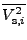of integrator i is then= Sin| fi|22 (18)
where

 | fi|22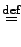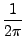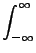Si(ω)| fi(jω)|2dω. (19)
Si(ω) is an input weighting function that is by default identical to 1. If the maximally allowed signal level at the outputs of the integrators is Vmax , the maximum allowed value for Sin is

 Smax, in =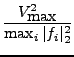. (20)
If H is the transfer function of the filter, the maximum output signal level of the filter is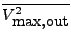= Smax, in| H(jω)|2dω = Smax, in| H|22. (21)

FA will use this assumption in determining maximal signal levels if the scale2 flag is set by

set: scale2Next: Sinusoid with fixed frequency Up: Maximum Signal Level Previous: Sinusoid with unknown frequency   Contents
2009-06-03